# Autoencoders for Wireless Communications

This example shows how to model an end-to-end communications system with an autoencoder to reliably transmit information bits over a wireless channel.

### Introduction

A traditional autoencoder is an unsupervised neural network that learns how to efficiently compress data, which is also called encoding. The autoencoder also learns how to reconstruct the data from the compressed representation such that the difference between the original data and the reconstructed data is minimal.

Traditional wireless communication systems are designed to provide reliable data transfer over a channel that impairs the transmitted signals. These systems have multiple components such as channel coding, modulation, equalization, synchronization, etc. Each component is optimized independently based on mathematical models that are simplified to arrive at closed form expressions. On the contrary, an autoencoder jointly optimizes the transmitter and the receiver as a whole. This joint optimization has the potential of providing a better performance than the traditional systems ,.

Traditional autoencoders are usually used to compress images, in other words remove redundancies in an image and reduce its dimension. A wireless communication system on the other hand uses channel coding and modulation techniques to add redundancy to the information bits. With this added redundancy, the system can recover the information bits that are impaired by the wireless channel. So, a wireless autoencoder actually adds redundancy and tries to minimize the number of errors in the received information for a given channel while learning to apply both channel coding and modulation in an unsupervised way.

### Basic Autoencoder System

The following is the block diagram of a wireless autoencoder system. The encoder (transmitter) first maps $\mathit{k}$ information bits into a message s such that $s\in \left\{1,\dots ,M\right\}$, where $M={2}^{k}$. Then message s is mapped to n real number to create $\text{x}=f\left(s\right)\in {\mathbb{R}}^{n}$. The last layer of the encoder imposes constraints on $\text{x}$ to further restrict the encoded symbols. The following are possible such constraints and are implemented using the normalization layer:

• Energy constraint: $‖x{‖}_{2}^{2}\le n$

• Average power constraint: $\mathbb{E}\left[|{x}_{i}{|}^{2}\right]\le 1,\forall i$Define the communication rate of this system as $R=k/n$ [bits/channel use], where (n,k) means that the system sends one of $M={2}^{k}$ messages using n channel uses. The channel impairs encoded (i.e. transmitted) symbols to generate $\text{y}\in {\mathbb{R}}^{n}$. The decoder (i.e. receiver) produces an estimate, $\underset{}{\overset{ˆ}{s}}$, of the transmitted message, $s$.

The input message is defined as a one-hot vector ${\text{1}}_{s}\in {\mathbb{R}}^{M}$, which is defined as a vector whose elements are all zeros except the ${\mathit{s}}^{\mathrm{th}}$ one. The channel is additive white Gaussian noise (AWGN) that adds noise to achieve a given energy per data bit to noise power density ratio, ${E}_{b}/{N}_{o}$.

The autoencoder maps $k$ data bits into $n$ channel uses, which results in an effective coding rate of $R=k/n$ data bits per channel use. Then, 2 channel uses are mapped into a symbol, which results in 2 channel uses per symbol. Map the channel uses per channel symbol value to the `BitsPerSymbol` parameter of the AWGN channel.

Define a (7,4) autoencoder network with energy normalization and a training ${E}_{b}/{N}_{o}$ of 3 dB. In , authors showed that two fully connected layers for both the encoder (transmitter) and the decoder (receiver) provides the best results with minimal complexity. Input layer (`featureInputLayer` (Deep Learning Toolbox)) accepts a one-hot vector of length M. The encoder has two fully connected layers (`fullyConnectedLayer` (Deep Learning Toolbox)). The first one has M inputs and M outputs and is followed by an ReLU layer (`reluLayer` (Deep Learning Toolbox)). The second fully connected layer has M inputs and n outputs and is followed by the normalization layer (helperAEWNormalizationLayer.m). The encoder layers are followed by the AWGN channel layer (helperAEWAWGNLayer.m). The output of the channel is passed to the decoder layers. The first decoder layer is a fully connected layer that has n inputs and M outputs and is followed by an ReLU layer. The second fully connected layer has M inputs and M outputs and is followed by a softmax layer (`softmaxLayer` (Deep Learning Toolbox)), which outputs the probability of each M symbols. The classification layer (`classificationLayer` (Deep Learning Toolbox)) outputs the most probable transmitted symbol from 0 to M-1.

```k = 4; % number of input bits M = 2^k; % number of possible input symbols n = 7; % number of channel uses EbNo = 3; % Eb/No in dB % Convert Eb/No to channel Eb/No values using the code rate R = k/n; EbNoChannel = EbNo + 10*log10(R); wirelessAutoencoder = [ featureInputLayer(M,"Name","One-hot input","Normalization","none") fullyConnectedLayer(M,"Name","fc_1") reluLayer("Name","relu_1") fullyConnectedLayer(n,"Name","fc_2") helperAEWNormalizationLayer("Method", "Energy", "Name", "wnorm") helperAEWAWGNLayer("Name","channel", ... "NoiseMethod","EbNo", ... "EbNo",EbNoChannel, ... "BitsPerSymbol",2, ... % channel use per channel symbol "SignalPower",1) fullyConnectedLayer(M,"Name","fc_3") reluLayer("Name","relu_2") fullyConnectedLayer(M,"Name","fc_4") softmaxLayer("Name","softmax") classificationLayer("Name","classoutput")]```
```wirelessAutoencoder = 11x1 Layer array with layers: 1 'One-hot input' Feature Input 16 features 2 'fc_1' Fully Connected 16 fully connected layer 3 'relu_1' ReLU ReLU 4 'fc_2' Fully Connected 7 fully connected layer 5 'wnorm' Wireless Normalization Energy normalization layer 6 'channel' AWGN Channel AWGN channel with EbNo = 0.56962 7 'fc_3' Fully Connected 16 fully connected layer 8 'relu_2' ReLU ReLU 9 'fc_4' Fully Connected 16 fully connected layer 10 'softmax' Softmax softmax 11 'classoutput' Classification Output crossentropyex ```

The `helperAEWTrainWirelessAutoencoder.m` function defines such a network based on the (n,k), normalization method and the ${E}_{b}/{N}_{o}$ values.

#### Train Autoencoder

Run the `helperAEWTrainWirelessAutoencoder.m` function to train a (2,2) autoencoder with energy normalization. This function uses the `trainingOptions` (Deep Learning Toolbox) function to select

• Initial learning rate of 0.08,

• Maximum epochs of 10,

• Minibatch size of 100*M,

• Piecewise learning schedule with drop period of 5 and drop factor of 0.1.

Then, the `helperAEWTrainWirelessAutoencoder.m` function runs the `trainNetwork` (Deep Learning Toolbox) function to train the autoencoder network with the selected options. Finally, this function separates the network into encoder and decoder parts. Encoder starts with the input layer and ends after the normalization layer. Decoder starts after the channel layer and ends with the classification layer. A feature input layer is added at the beginning of the decoder.

Train the autoencoder with an ${E}_{b}/{N}_{o}$ value that is low enough to result in some errors but not too low such that the training algorithm cannot extract any useful information from the received symbols, y. Set ${E}_{b}/{N}_{o}$ to 3 dB.

```n = 2; % number of channel uses k = 2; % number of input bits EbNo = 3; % dB normalization = "Energy"; % Normalization "Energy" | "Average power" [txNet(1),rxNet(1),infoTemp,wirelessAutoEncoder(1)] = ... helperAEWTrainWirelessAutoencoder(n,k,normalization,EbNo); infoTemp.n = n; infoTemp.k = k; infoTemp.EbNo = EbNo; infoTemp.Normalization = normalization; info = infoTemp;```

Plot the training progress. The validation accuracy quickly reaches more than 90% while the validation loss keeps slowly decreasing. This behavior shows that the training ${E}_{b}/{N}_{o}$ value was low enough to cause some errors but not too low to avoid convergence. For definitions of validation accuracy and validation loss, see Monitor Deep Learning Training Progress (Deep Learning Toolbox) section.

```figure helperAEWPlotTrainingPerformance(info(1))```Use the plot object function of the trained network objects to show the layer graphs of the full autoencoder, the encoder network, i.e. the transmitter, and the decoder network, i.e. the receiver.

```figure tiledlayout(2,2) nexttile([2 1]) plot(wirelessAutoEncoder(1)) title('Autoencoder') nexttile plot(txNet(1)) title('Encoder/Tx') nexttile plot(rxNet(1)) title('Decoder/Rx')```### Simulate BLER Performance

Simulate the block error rate (BLER) performance of the (2,2) autoencoder. Set up simulation parameters.

```simParams.EbNoVec = 0:0.5:8; simParams.MinNumErrors = 10; simParams.MaxNumFrames = 300; simParams.NumSymbolsPerFrame = 10000; simParams.SignalPower = 1;```

Generate random integers in the [0 $M$-1] range that represents $k$ random information bits. Encode these information bits into complex symbols with `helperAEWEncode` function. `The helperAEWEncode` function runs the encoder part of the autoencoder then maps the real valued $\text{x}$ vector into a complex valued ${x}_{c}$ vector such that the odd and even elements are mapped into the in-phase and the quadrature component of a complex symbol, respectively, where ${\text{x}}_{c}=\text{x}\left(1:2:end\right)+j\text{x}\left(2:2:end\right)$. In other words, treat the $\text{x}$ array as an interleaved complex array.

Pass the complex symbols through an AWGN channel. Decode the channel impaired complex symbols with the `helperAEWDecode` function. The following code runs the simulation for each ${E}_{b}/{N}_{o}$ point for at least 10 block errors. To obtain more accurate results, increase minimum number of errors to at least 100. If Parallel Computing Toolbox™ is installed and a license is available, uncomment the `parfor` line to run the simulations on a parallel pool.

Plot the constellation learned by the autoencoder to send symbols through the AWGN channel together with the received constellation. For a (2,2) configuration, autoencoder learns a QPSK ($M={2}^{k}=4$) constellation with a phase rotation.

```R = k/n; EbNoChannelVec = simParams.EbNoVec + 10*log10(R); M = 2^k; txConst = comm.ConstellationDiagram(ShowReferenceConstellation=false, ... ShowLegend=true, ChannelNames={'Tx Constellation'}); rxConst = comm.ConstellationDiagram(ShowReferenceConstellation=false, ... ShowLegend=true, ChannelNames={'Rx Constellation'}); BLER = zeros(size(EbNoChannelVec)); %parfor trainingEbNoIdx = 1:length(EbNoChannelVec) for trainingEbNoIdx = 1:length(EbNoChannelVec) EbNo = EbNoChannelVec(trainingEbNoIdx); chan = comm.AWGNChannel("BitsPerSymbol",2, ... "EbNo", EbNo, "SamplesPerSymbol", 1, "SignalPower", 1); numBlockErrors = 0; frameCnt = 0; while (numBlockErrors < simParams.MinNumErrors) ... && (frameCnt < simParams.MaxNumFrames) d = randi([0 M-1],simParams.NumSymbolsPerFrame,1); % Random information bits x = helperAEWEncode(d,txNet(1)); % Encoder txConst(x) y = chan(x); % Channel rxConst(y) dHat = helperAEWDecode(y,rxNet(1)); % Decoder numBlockErrors = numBlockErrors + sum(d ~= dHat); frameCnt = frameCnt + 1; end BLER(trainingEbNoIdx) = numBlockErrors / (frameCnt*simParams.NumSymbolsPerFrame); end```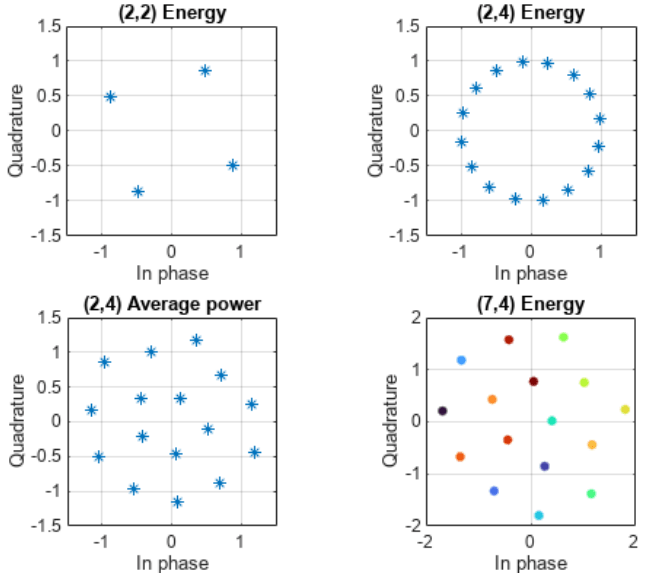Compare the results with that of an uncoded QPSK system with block length n=2. For this n value, the autoencoder gets the same BLER as an uncoded QPSK system.

```figure semilogy(simParams.EbNoVec,BLER,'-') hold on % Calculate uncoded block error rate (R=k/n=1) pskBLER = 1-(1-berawgn(EbNoChannelVec,'psk',2^k,'nondiff')).^n; semilogy(simParams.EbNoVec,pskBLER,'--') hold off ylim([1e-4 1]) grid on xlabel('E_b/N_o (dB)') ylabel('BLER') legend(sprintf('AE (%d,%d)',n,k),sprintf('QPSK (%d,%d)',n,k))```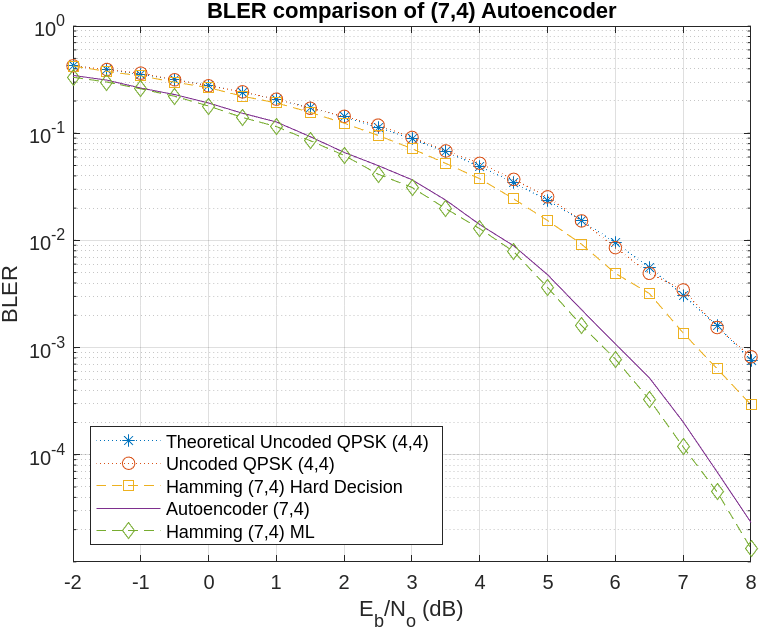The well formed constellation together with the BLER results show that training for 10 epochs is enough to get a satisfactory convergence.

### Compare Constellation Diagrams

Compare learned constellations of several autoencoders normalized to unit energy and unit average power. Train (2,4) autoencoder normalized to unit energy.

```n = 2; % number of channel uses k = 4; % number of input bits EbNo = 9; % dB normalization = "Energy"; [txNet(2),rxNet(2),infoTemp,wirelessAutoEncoder(2)] = ... helperAEWTrainWirelessAutoencoder(n,k,normalization,EbNo); infoTemp.n = n; infoTemp.k = k; infoTemp.EbNo = EbNo; infoTemp.Normalization = normalization; info(2) = infoTemp;```

Train (2,4) autoencoder normalized to unit average power.

```n = 2; % number of channel uses k = 4; % number of input bits EbNo = 6; % dB normalization = "Average power"; [txNet(3),rxNet(3),infoTemp,wirelessAutoEncoder(3)] = ... helperAEWTrainWirelessAutoencoder(n,k,normalization,EbNo); infoTemp.n = n; infoTemp.k = k; infoTemp.EbNo = EbNo; infoTemp.Normalization = normalization; info(3) = infoTemp;```

Train (7,4) autoencoder normalized to unit energy.

```n = 7; % number of channel uses k = 4; % number of input bits EbNo = 3; % dB normalization = "Energy"; [txNet(4),rxNet(4),infoTemp,wirelessAutoEncoder(4)] = ... helperAEWTrainWirelessAutoencoder(n,k,normalization,EbNo); infoTemp.n = n; infoTemp.k = k; infoTemp.EbNo = EbNo; infoTemp.Normalization = normalization; info(4) = infoTemp;```

Plot the constellation using the `helperAEWPlotConstellation.m` function. The trained (2,2) autoencoder converges on a QPSK constellation with a phase shift as the optimal constellation for the channel conditions experienced. The (2,4) autoencoder with energy normalization converges to a 16PSK constellation with a phase shift. Note that, energy normalization forces every symbol to have unit energy and places the symbols on the unit circle. Given this constraint, best constellation is a PSK constellation with equal angular distance between symbols. The (2,4) autoencoder with average power normalization converges to a three-tier constellation of 1-6-9 symbols. Average power normalization forces the symbols to have unity average power over time. This constraint results in an APSK constellation, which is different than the conventional QAM or APSK schemes. Note that, this network configuration may also converge to a two-tier constellation with 7-9 symbols based on the random initial condition used during training. The last plot shows the 2-D mapping of the 7-D constellation generated by the (7,4) autoencoder with energy constraint. 2-D mapping is obtained using the t-Distributed Stochastic Neighbor Embedding (t-SNE) method (see `tsne` (Statistics and Machine Learning Toolbox) function).

```figure subplot(2,2,1) helperAEWPlotConstellation(txNet(1)) title(sprintf('(%d,%d) %s',info(1).n,info(1).k,info(1).Normalization)) subplot(2,2,2) helperAEWPlotConstellation(txNet(2)) title(sprintf('(%d,%d) %s',info(2).n,info(2).k,info(2).Normalization)) subplot(2,2,3) helperAEWPlotConstellation(txNet(3)) title(sprintf('(%d,%d) %s',info(3).n,info(3).k,info(3).Normalization)) subplot(2,2,4) helperAEWPlotConstellation(txNet(4),'t-sne') title(sprintf('(%d,%d) %s',info(4).n,info(4).k,info(4).Normalization))```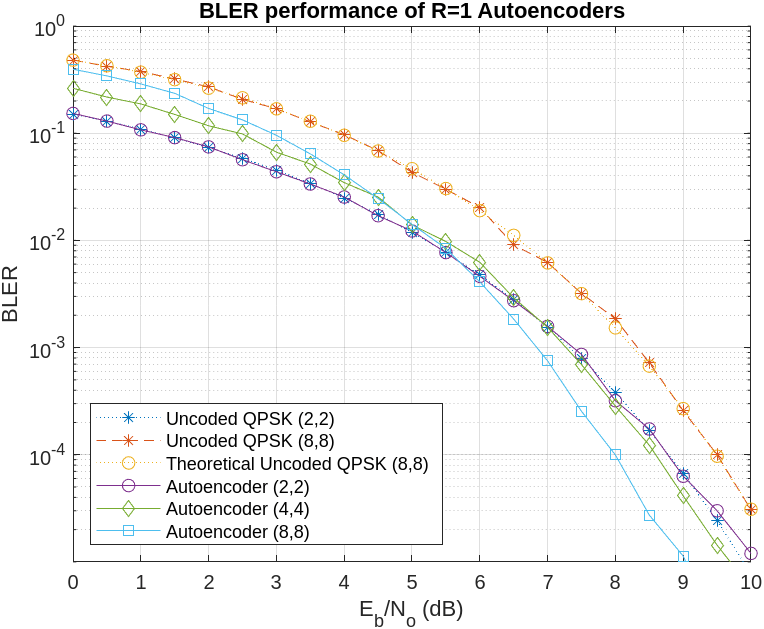### Compare BLER Performance of Autoencoders with Coded and Uncoded QPSK

Simulate the BLER performance of a (7,4) autoencoder with that of (7,4) Hamming code with QPSK modulation for both hard decision and maximum likelihood (ML) decoding. Use uncoded (4,4) QPSK as a baseline. (4,4) uncoded QPSK is basically a QPSK modulated system that sends blocks of 4 bits and measures BLER. The data for the following figures is obtained using helperAEWSimulateBLER.mlx and helperAEWPrepareAutoencoders.mlx files.

```load codedBLERResults.mat figure qpsk44BLERTh = 1-(1-berawgn(simParams.EbNoVec,'psk',4,'nondiff')).^4; semilogy(simParams.EbNoVec,qpsk44BLERTh,':*') hold on semilogy(simParams.EbNoVec,qpsk44BLER,':o') semilogy(simParams.EbNoVec,hammingHard74BLER,'--s') semilogy(simParams.EbNoVec,ae74eBLER,'-') semilogy(simParams.EbNoVec,hammingML74BLER,'--d') hold off ylim([1e-5 1]) grid on xlabel('E_b/N_o (dB)') ylabel('BLER') legend('Theoretical Uncoded QPSK (4,4)','Uncoded QPSK (4,4)','Hamming (7,4) Hard Decision', ... 'Autoencoder (7,4)','Hamming (7,4) ML','Location','southwest') title('BLER comparison of (7,4) Autoencoder')```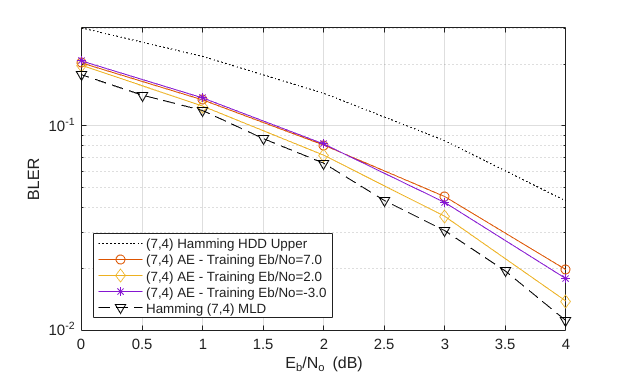As expected, hard decision (7,4) Hamming code with QPSK modulation provides about 0.6 dB ${E}_{b}/{N}_{o}$ advantage over uncoded QPSK, while the ML decoding of (7,4) Hamming code with QPSK modulation provides another 1.5 dB advantage for a BLER of $1{0}^{-3}$. The (7,4) autoencoder BLER performance approaches the ML decoding of (7,4) Hamming code, when trained with 3 dB ${E}_{b}/{N}_{o}$. This BLER performance shows that the autoencoder is able to learn not only modulation but also channel coding to achieve a coding gain of about 2 dB for a coding rate of R=4/7.

Next, simulate the BLER performance of autoencoders with R=1 with that of uncoded QPSK systems. Use uncoded (2,2) and (8,8) QPSK as baselines. Compare BLER performance of these systems with that of (2,2), (4,4) and (8,8) autoencoders.

```load uncodedBLERResults.mat qpsk22BLERTh = 1-(1-berawgn(simParams.EbNoVec,'psk',4,'nondiff')).^2; semilogy(simParams.EbNoVec,qpsk22BLERTh,':*') hold on semilogy(simParams.EbNoVec,qpsk88BLER,'--*') qpsk88BLERTh = 1-(1-berawgn(simParams.EbNoVec,'psk',4,'nondiff')).^8; semilogy(simParams.EbNoVec,qpsk88BLERTh,':o') semilogy(simParams.EbNoVec,ae22eBLER,'-o') semilogy(simParams.EbNoVec,ae44eBLER,'-d') semilogy(simParams.EbNoVec,ae88eBLER,'-s') hold off ylim([1e-5 1]) grid on xlabel('E_b/N_o (dB)') ylabel('BLER') legend('Uncoded QPSK (2,2)','Uncoded QPSK (8,8)','Theoretical Uncoded QPSK (8,8)', ... 'Autoencoder (2,2)','Autoencoder (4,4)','Autoencoder (8,8)','Location','southwest') title('BLER performance of R=1 Autoencoders')```Bit error rate of QPSK is the same for both (8,8) and (2,2) cases. However, the BLER depends on the block length, $n$, and gets worse as $n$ increases as given by $BLER=1-\left(1-BER{\right)}^{n}$. As expected, BLER performance of (8,8) QPSK is worse than the (2,2) QPSK system. The BLER performance of (2,2) autoencoder matches the BLER performance of (2,2) QPSK. On the other hand, (4,4) and (8,8) autoencoders optimize the channel coder and the constellation jointly to obtain a coding gain with respect to the corresponding uncoded QPSK systems.

### Effect of Training Eb/No on BLER Performance

Train the (7,4) autoencoder with energy normalization under different ${E}_{b}/{N}_{o}$ values and compare the BLER performance. To extend the BLER curve, set simParams.EbNoVec to -2:0.5:8.

```n = 7; k = 4; normalization = 'Energy'; traningEbNoVec = -3:5:7; simParams.EbNoVec = 0:4; for trainingEbNoIdx = 1:length(traningEbNoVec) trainingEbNo = traningEbNoVec(trainingEbNoIdx); [txNetVec{trainingEbNoIdx},rxNetVec{trainingEbNoIdx},infoVec{trainingEbNoIdx},trainedNetVec{trainingEbNoIdx}] = ... helperAEWTrainWirelessAutoencoder(n,k,normalization,trainingEbNo); %#ok<SAGROW> BLERVec{trainingEbNoIdx} = helperAEWAutoencoderBLER(txNetVec{trainingEbNoIdx},rxNetVec{trainingEbNoIdx},simParams); %#ok<SAGROW> end```

Plot the BLER performance together with theoretical upper bound for hard decision decoded Hamming (7,4) code and simulated BLER of maximum likelihood decoded (MLD) Hamming (7,4) code. The BLER performance of the (7,4) autoencoder gets closer to the Hamming (7,4) code with MLD as the training ${E}_{b}/{N}_{o}$ decreases from 10 dB to 1 dB, at which point it almost matches the MLD Hamming (7,4) code.

```berHamming = bercoding(simParams.EbNoVec,'hamming','hard',n); blerHamming = 1-(1-berHamming).^k; hammingBLER = load('codedBLERResults'); figure semilogy(simParams.EbNoVec,blerHamming,':k') legendStr = sprintf('(%d,%d) Hamming HDD Upper',n,k); hold on linespec = {'-*','-d','-o','-s',}; for trainingEbNoIdx=length(traningEbNoVec):-1:1 semilogy(simParams.EbNoVec,BLERVec{trainingEbNoIdx},linespec{trainingEbNoIdx}) legendStr = [legendStr {sprintf('(%d,%d) AE - Training Eb/No=%1.1f', ... n,k,traningEbNoVec(trainingEbNoIdx))}]; %#ok<AGROW> end semilogy(hammingBLER.simParams.EbNoVec,hammingBLER.hammingML74BLER,'--vk') legendStr = [legendStr {'Hamming (7,4) MLD'}]; hold off xlim([min(simParams.EbNoVec) max(simParams.EbNoVec)]) grid on xlabel('E_b/N_o (dB)') ylabel('BLER') legend(legendStr{:},'location','southwest')```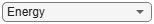### Conclusions and Further Exploration

The BLER results show that it is possible for autoencoders to learn joint coding and modulation schemes in an unsupervised way. It is even possible to train an autoencoder with R=1 to obtain a coding gain as compared to traditional methods. The example also shows the effect of hyperparameters such as ${E}_{b}/{N}_{o}$ on the BLER performance.

The results are obtained using the following default settings for training and BLER simulations:

```trainParams.Plots = 'none'; trainParams.Verbose = false; trainParams.MaxEpochs = 10; trainParams.InitialLearnRate = 0.08; trainParams.LearnRateSchedule = 'piecewise'; trainParams.LearnRateDropPeriod = 5; trainParams.LearnRateDropFactor = 0.1; trainParams.MiniBatchSize = 100*2^k; simParams.EbNoVec = -2:0.5:8; simParams.MinNumErrors = 100; simParams.MaxNumFrames = 300; simParams.NumSymbolsPerFrame = 10000; simParams.SignalPower = 1;```

Vary these parameters to train different autoencoders and test their BLER performance. Experiment with different n, k, normalization and ${E}_{b}/{N}_{o}$ values. See the help for helperAEWTrainWirelessAutoencoder.m, helperAEWPrepareAutoencoders.mlx and helperAEWAutoencoderBLER.m for more information.

### References

 T. O’Shea and J. Hoydis, "An Introduction to Deep Learning for the Physical Layer," in IEEE Transactions on Cognitive Communications and Networking, vol. 3, no. 4, pp. 563-575, Dec. 2017, doi: 10.1109/TCCN.2017.2758370.

 S. Dörner, S. Cammerer, J. Hoydis and S. t. Brink, "Deep Learning Based Communication Over the Air," in IEEE Journal of Selected Topics in Signal Processing, vol. 12, no. 1, pp. 132-143, Feb. 2018, doi: 10.1109/JSTSP.2017.2784180.